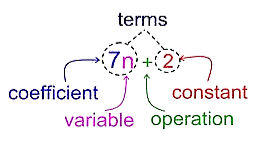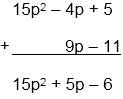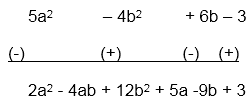×#### Thank you for registering.

One of our academic counsellors will contact you within 1 working day.

Click to Chat

1800-1023-196

+91-120-4616500

CART 0

• 0

MY CART (5)

Use Coupon: CART20 and get 20% off on all online Study Material

ITEM
DETAILS
MRP
DISCOUNT
FINAL PRICE
Total Price: Rs.

There are no items in this cart.
Continue Shopping• Complete JEE Main/Advanced Course and Test Series
• OFFERED PRICE: Rs. 15,900
• View Details

```Revision Notes on Algebraic Expressions and Identities

Algebraic Expression

Any mathematical expression which consists of numbers, variables and operations are called Algebraic Expression.1. Terms

Every expression is separated by an operation which is called Terms. Like 7n and 2 are the two terms in the above figure.

2. Factors

Every term is formed by the product of the factors.7n is the product of 7 and n which are the factors of 7n.

3. Coefficient

The number placed before the variable or the numerical factor of the term is called Coefficient of that variable.7 is the numerical factor of 7n so 7 is coefficient here.

4. Variable

Any letter like x, y etc. are called Variables. The variable in the above figure is n.

5. Operations

Addition, subtraction etc. are the operations which separate each term.

6. Constant

The number without any variable is constant. 2 is constant here.

Number Line and an Expression

An expression can be represented on the number line.

Example

How to represent x + 5 and x – 5 on the number line?

Solution:

First, mark the distance x and then x + 5 will be 5 unit to the right of x.In the case of x – 5 we will start from the right and move towards the negative side. x – 5 will be 5 units to the left of x.

Monomials, Binomials and PolynomialsExpressions
Meaning
Example

Monomial

The algebraic expression having only one term.

5x2

Binomial

The algebraic expression having two terms.

5x2 + 2y

Trinomial

The algebraic expression having three terms.

5x2 + 2y + 9xy

Polynomial

The algebraic expression having one or more terms with the variable having non-negative integers as an exponent.

5x2 + 2y + 9xy + 4 and all the above expressions are also polynomial.

Like and Unlike Terms

Terms having the same variable are called Like Terms.

Examples of Like Terms

2x and -9x

24xy and 5yx

6x2 and 12x2

The terms having different variable are called, Unlike Terms.

Examples of Unlike Terms

2x and - 9y

24xy and 5pq

6x2 and 12y2

Addition and Subtraction of Algebraic Expressions

Steps to add or Subtract Algebraic Expression

First of all, we have to write the algebraic expressions in different rows in such a way that the like terms come in the same column.

If any term of the same variable is not there in another expression then write is as it is in the solution.

Example

Add 15p2 – 4p + 5 and 9p – 11

Solution:

Write down the expressions in separate rows with like terms in the same column and add.Example

Subtract 5a2 – 4b2 + 6b – 3 from 7a2 – 4ab + 8b2 + 5a – 3b.

Solution:

For subtraction also write the expressions in different rows. But to subtract we have to change their signs from negative to positive and vice versa.Multiplication of Algebraic Expressions

While multiplying we need to take care of some points about the multiplication of like and unlike terms.

1. Multiplication of Like Terms

The coefficients will get multiplied.

The power will not get multiplied but the resultant variable will be the addition of the individual powers.

Example

The product of 4x and 3x will be 12x2.

The product of 5x, 3x and 4x will be 60x3.

2. Multiplication of Unlike Terms

The coefficients will get multiplied.

The power will remain the same if the variable is different.

If some of the variables are the same then their powers will be added.

Example

The product of 2p and 3q will be 6pq

The product of 2x2y, 3x and 9 will  be 54x3y

Multiplying a Monomial by a Monomial

1. Multiplying Two Monomials

While multiplying two polynomials the resultant variable will come by

The coefficient of product = Coefficient of the first monomial × Coefficient of the second monomial

The algebraic factor of product = Algebraic factor of the first monomial × Algebraic factor of the second monomial.

Example

25y × 3xy = 125xy2

2. Multiplying Three or More Monomials

While multiplying three or more monomial the criterion will remain the same.

Example

4xy × 5x2y2 × 6x3y3 = (4xy × 5x2y2) × 6x3y3

= 20x3y3 × 6x3y3

= 120x3y3 × x3y3

= 120 (x3 × x3) × (y3 × y3)

= 120x6 × y6

= 120x6y6

We can do it in other way also

4xy × 5x2y2 × 6x3 y3

= (4 × 5 × 6) × (x × x2 × x3) × (y × y2 × y3)

= 120 x6y6

Multiplying a Monomial by a Polynomial

1. Multiplying a Monomial by a Binomial

To multiply a monomial with a binomial we have to multiply the monomial with each term of the binomial.

Example

Multiplication of 8 and (x + y) will be (8x + 8y).

Multiplication of 3x and (4y + 7) will be (12xy + 21x).

Multiplication of 7x3 and (2x4 + y4) will be (14x7+ 7x3y4).

2. Multiplication of Monomial by a trinomial

This is also the same as above.

Example

Multiplication of 8 and (x + y + z) will be (8x + 8y + 8z).

Multiplication of 4x and (2x + y + z) will be (8x2 + 4xy + 4xz).

Multiplication of 7x3 and (2x4+ y4+ 2) will be (14x7 + 7x3y4 + 14x3).

Multiplying a Polynomial by a Polynomial

1. Multiplying a Binomial by a Binomial

We use the distributive law of multiplication in this case. Multiply each term of a binomial with every term of another binomial. After multiplying the polynomials we have to look for the like terms and combine them.

Example

Simplify (3a + 4b) × (2a + 3b)

Solution:

(3a + 4b) × (2a + 3b)

= 3a × (2a + 3b) + 4b × (2a + 3b)    [distributive law]

= (3a × 2a) + (3a × 3b) + (4b × 2a) + (4b × 3b)

= 6 a2 + 9ab + 8ba + 12b2

= 6 a2 + 17ab + 12b2     [Since ba = ab]

2. Multiplying a Binomial by a Trinomial

In this also we have to multiply each term of the binomial with every term of trinomial.

Example

Simplify (p + q) (2p – 3q + r) – (2p – 3q) r.

Solution:

We have a binomial (p + q) and one trinomial (2p – 3q + r)

(p + q) (2p – 3q + r)

= p(2p – 3q + r) + q (2p – 3q + r)

= 2p2 – 3pq + pr + 2pq – 3q2 + qr

= 2p2 – pq – 3q2 + qr + pr    (–3pq and 2pq are like terms)

(2p – 3q) r = 2pr – 3qr

Therefore,

(p + q) (2p – 3q + r) – (2p – 3q) r

= 2p2 – pq – 3q2 + qr + pr – (2pr – 3qr)

= 2p2 – pq – 3q2 + qr + pr – 2pr + 3qr

= 2p2 – pq – 3q2 + (qr + 3qr) + (pr – 2pr)

= 2p2 – 3q2 – pq + 4qr – pr

Identities

An identity is an equality which is true for every value of the variable but an equation is true for only some of the values of the variables.

So an equation is not an identity.

Like, x2 = 1, is valid if x is 1 but is not true if x is 2.so it is an equation but not an identity.

Some of the Standard Identities

(a + b)2 = a2 + 2ab + b2

(a - b)2 = a2 – 2ab + b2

a2 – b2 = (a + b) (a - b)

(x + a) (x + b) = x2 + (a + b)x + ab

(a + b + c)2 = a2 + b2 + c2 + 2ab + 2bc + 2ca

These identities are useful in carrying out squares and products of algebraic expressions. They give alternative methods to calculate products of numbers and so on.

Applying Identities

Example

(4x – 3y)2

= (4x)2 – 2(4x) (3y) + (3y)2

= 16x2 – 24xy + 9y2

Example

Use the Identity (x + a) (x + b) = x2 + (a + b) x + ab to find the value of 501 × 502

Solution:

501 × 502

= (500 + 1) × (500 + 2)

= 5002 + (1 + 2) × 500 + 1 × 2

= 250000 + 1500 + 2

= 251502
```### Course Features

• 728 Video Lectures
• Revision Notes
• Previous Year Papers
• Mind Map
• Study Planner
• NCERT Solutions
• Discussion Forum
• Test paper with Video Solution